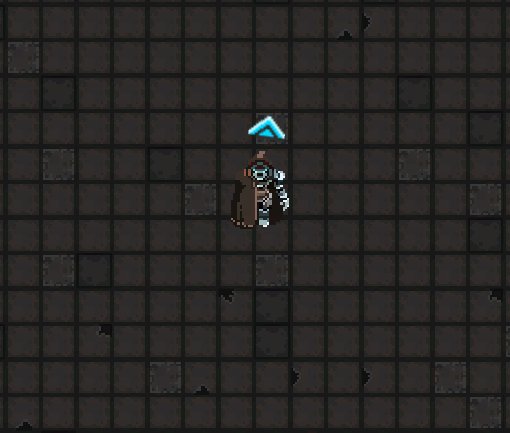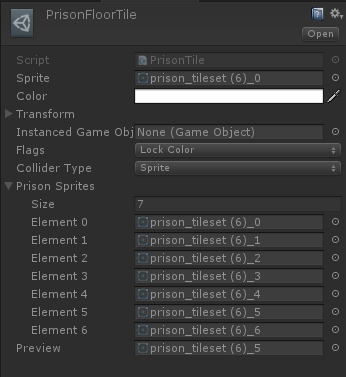Creating Randomize Tiles for Unity 2D Tilemaps

This is how we create custom floor tiles to lay random floor sprites.

Posted by on - Basic Mapping/Technical

I want to go over how I'm laying down random floor tiles in some of the levels in Memory Breach.

Outcome:You can see how there are different floor tiles scattered about, but I definitely did not take time to "randomly" lay down different tiles to accomplish this.

By using Unity's base `Tile` class, I can create a special tile that using logic to lay down sprites.

Code:

``````public class PrisonTile : Tile {

[SerializeField]
private Sprite[] prisonSprites;

[SerializeField]
private Sprite preview;

public override void GetTileData(Vector3Int position, ITilemap tilemap, ref TileData tileData)
{
var floor2 = 15.0f;
var floor3 = 30.0f;
var floor4 = 32.0f;
var floor5 = 34.0f;
var floor6 = 36.0f;
var floor7 = 38.0f;

var choosen = Random.Range(0.0f, 300.0f);

if (choosen >= 0f && choosen < floor2)
{
tileData.sprite = prisonSprites;
}
else if (choosen >= floor2 && choosen < floor3)
{
tileData.sprite = prisonSprites;
}
else if (choosen >= floor3 && choosen < floor4)
{
tileData.sprite = prisonSprites;
}
else if (choosen >= floor4 && choosen < floor5)
{
tileData.sprite = prisonSprites;
}
else if (choosen >= floor5 && choosen < floor6)
{
tileData.sprite = prisonSprites;
}
else if (choosen >= floor6 && choosen <= floor7)
{
tileData.sprite = prisonSprites;
}else
{
tileData.sprite = prisonSprites;
}

}

#if UNITY_EDITOR
public static void CreatePrisonTile()
{
string path = EditorUtility.SaveFilePanelInProject("Save Prisontile", "New PrisonTile", "asset", "Save prison tile", "Assets");
if(path == "")
{
return;
}

AssetDatabase.CreateAsset(ScriptableObject.CreateInstance<PrisonTile>(), path);
}
#endif
}``````

Now there are many aspects to this class, but overall its pretty simple. There are two parts I want to go over specifically:

``private Sprite[] prisonSprites``

and

``public override void GetTileData``

private Sprite[] prisonSprites holds a list of sprites that we pick from when we randomly lay down a sprite. As you can see in the Inspector below, I just state how many sprites will be in the list and then assign the sprites in the list item spots.Lets move to public override void GetTileData. This is where we actually write some logic to pick which sprite to lay down.

``````var floor2 = 15.0f;
var floor3 = 30.0f;
var floor4 = 32.0f;
var floor5 = 34.0f;
var floor6 = 36.0f;
var floor7 = 38.0f;``````

There are total of 7 different floor sprites I pick from. Here we only see 2 - 7 and this is because the first sprite is our default sprite to lay down. Floor 2 and 3 both have a 5% chance of being placed and Floors 4 - 7 only have a 0.6% chance. Lets look at how we determine the odds of a tile being placed.

``var choosen = Random.Range(0.0f, 300.0f);``

We choose a random float number between 0 and 300. Now look at the if statement.

The first condition states

``if (choosen >= 0f && choosen < floor2)``

This means if choosen is greater than or equals 0 and is less than floor2, aka 15.0, then we can place a Floor 2 tile.

Note that 15 divided by 300 = 0.05 or 5%. If we choose 0 to 100 then it would be 15%. I did originally start with 0 to 100 to keep it simple at first, then modified values to hone in what I thought looked good.

We do this type of comparison for each of the non-main tiles. If choosen is greater than or equal to previous floor tile value and is less than current floor tile value, then lay down current floor tile.

If no condition is met, then lay down main floor tile.